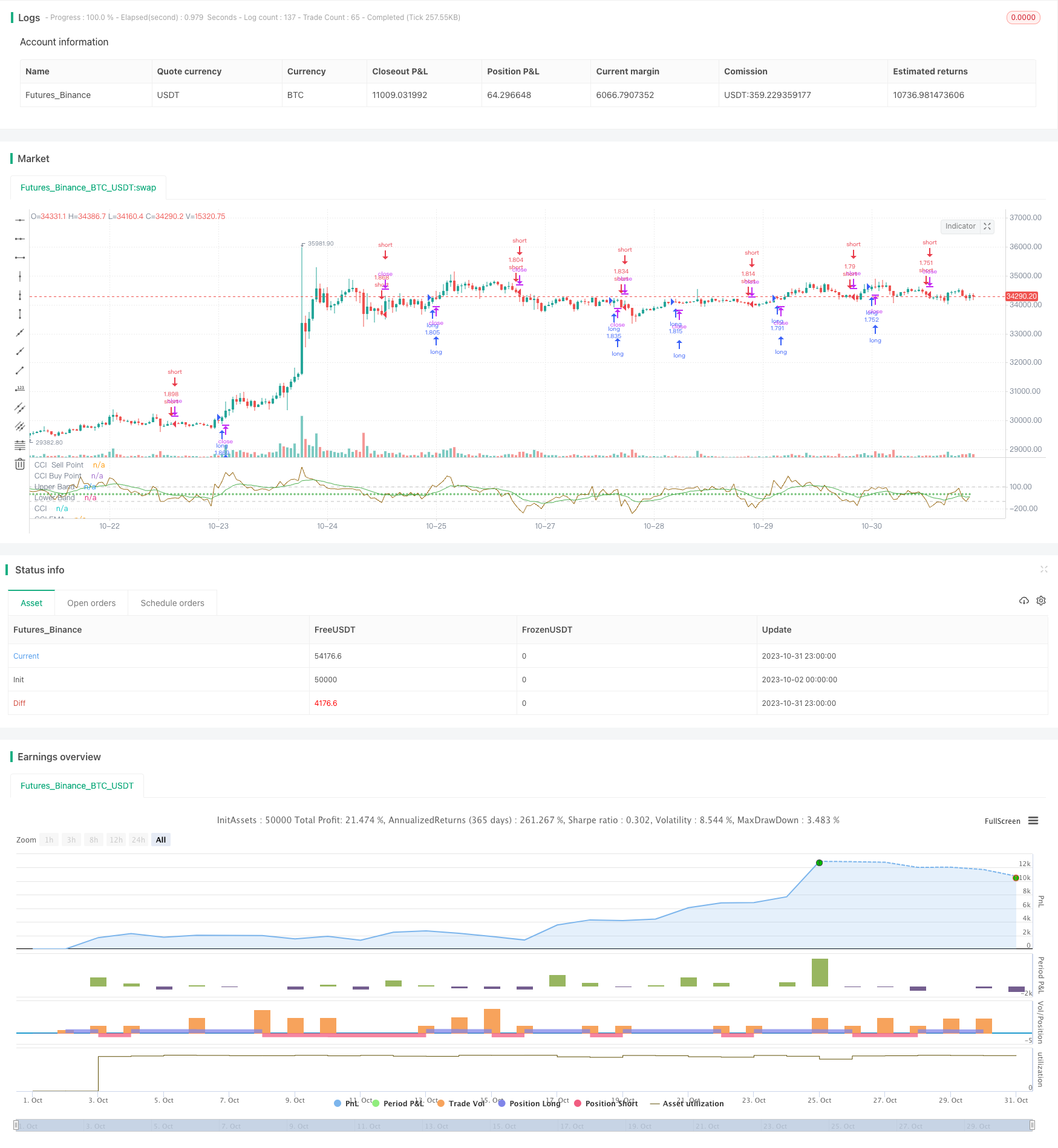# CCI and EMA Trend Following Trading Strategy

Author: ChaoZhang, Date: 2023-11-02 15:17:22
Tags:## Overview

The core idea of this strategy is to use the CCI indicator to determine the market trend direction and use the EMA indicator to smooth the CCI to implement trend following trading. Go long when the CCI crosses above the buy point and go short when the CCI crosses below the sell point to follow the market trend.

## Strategy Logic

1. Calculate the CCI indicator. The CCI indicator judges whether the current stock price is overbought or oversold based on the degree of deviation from the 20-day moving average. The formula is: (typical price - 20D SMA) / (0.015 * 20D TP standard deviation).

2. Smooth the CCI indicator with an EMA to get a CCI-EMA curve, which reduces the fluctuation of the CCI and makes the signal clearer.

3. Set the buy and sell points for CCI. Go long when CCI-EMA crosses above the buy point, and go short when CCI-EMA crosses below the sell point.

4. Hold the position until CCI-EMA touches the buy or sell point again to close the position.

1. Using CCI to determine market trend direction combined with EMA to filter false signals can effectively follow market trends.

2. CCI is sensitive to price anomalies and can quickly capture trend reversals. EMA reduces false signals. Used together, they can seize opportunities at the beginning of trends.

3. Trend following strategies minimize transactions, reduce trading costs and slippage.

4. The backtest results are decent, giving the strategy some practical viability.

## Risk Analysis

1. CCI can be overly sensitive to curves and EMA cannot completely filter all false signals, some false signals remain.

2. Pure trend following strategies are prone to losses when trends consolidate or reverse. Trend judgment indicators should be used.

3. Mechanical trading systems cannot flexibly adjust parameters based on markets. Over optimization is a risk.

4. Limited backtest data cannot fully reflect live performance. Parameters should be adjusted carefully and stops managed strictly.

## Optimization Directions

1. Optimize CCI parameters by testing different length periods.

2. Optimize EMA parameters to find the optimal EMA period.

3. Test different buy/sell point combinations to find the optimal parameters.

4. Incorporate other indicators to determine trend reversal and set stop losses.

5. Add auto parameter optimization to find the optimal parameters for different products.

## Summary

Overall this is a relatively simple trend following trading strategy. It uses CCI to determine trend direction and is sensitive to price changes, combined with EMA filtering to generate signals. The strategy has some advantages but also risks to note. Through parameter optimization and using other indicators, the stability and live performance can be further improved. Overall it provides a simple and reliable trend following template for quant trading.

```/*backtest
start: 2023-10-02 00:00:00
end: 2023-11-01 00:00:00
period: 1h
basePeriod: 15m
exchanges: [{"eid":"Futures_Binance","currency":"BTC_USDT"}]
*/

//@version=4
strategy("CCI with EMA Strategy", overlay=false, pyramiding=1, default_qty_type= strategy.percent_of_equity, default_qty_value = 100, calc_on_order_fills=false, slippage=0,commission_type=strategy.commission.percent,commission_value=0.07)

length = input(20, minval=1)
src = input(close, title="Source")
cciSellPoint = input(0, title = 'CCI Sell Point', type = input.integer)
lengthcci = input(12,"length cci ema", minval=1)

ma = sma(src, length)
cci = (src - ma) / (0.015 * dev(src, length))
cciema=ema(cci,lengthcci)
plot(cci, "CCI", color=#996A15)
plot(cciSellPoint, title = 'CCI  Sell Point', color = color.red, linewidth = 1, style = plot.style_cross, transp = 35)
plot(cciBuyPoint, title = 'CCI Buy Point', color = color.green, linewidth = 1, style = plot.style_cross, transp = 35)
plot(cciema, title = 'CCI EMA', color = color.green, linewidth = 1, transp = 35)
band1 = hline(100, "Upper Band", color=#C0C0C0, linestyle=hline.style_dashed)
band0 = hline(-100, "Lower Band", color=#C0C0C0, linestyle=hline.style_dashed)
fill(band1, band0, color=#9C6E1B, title="Background")